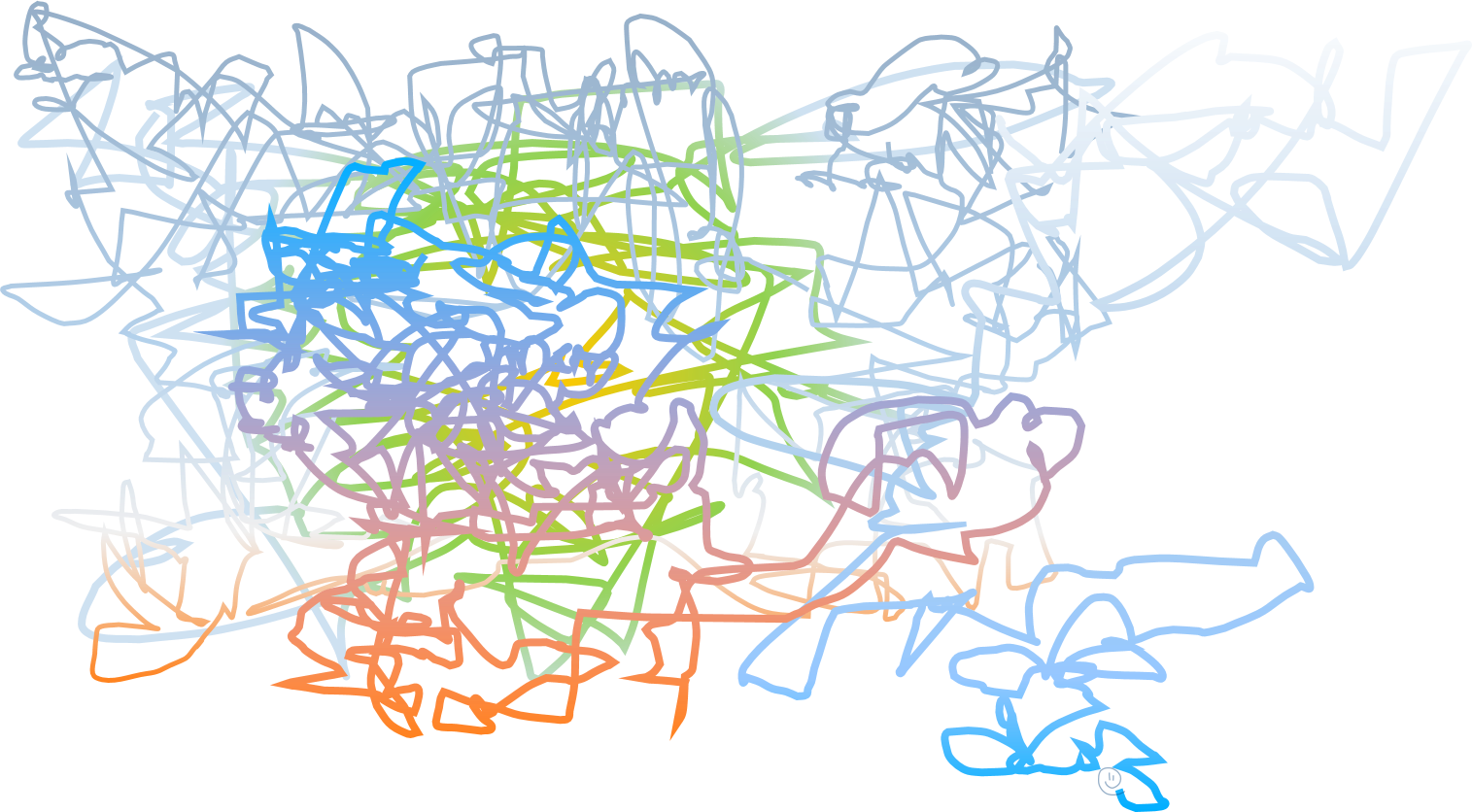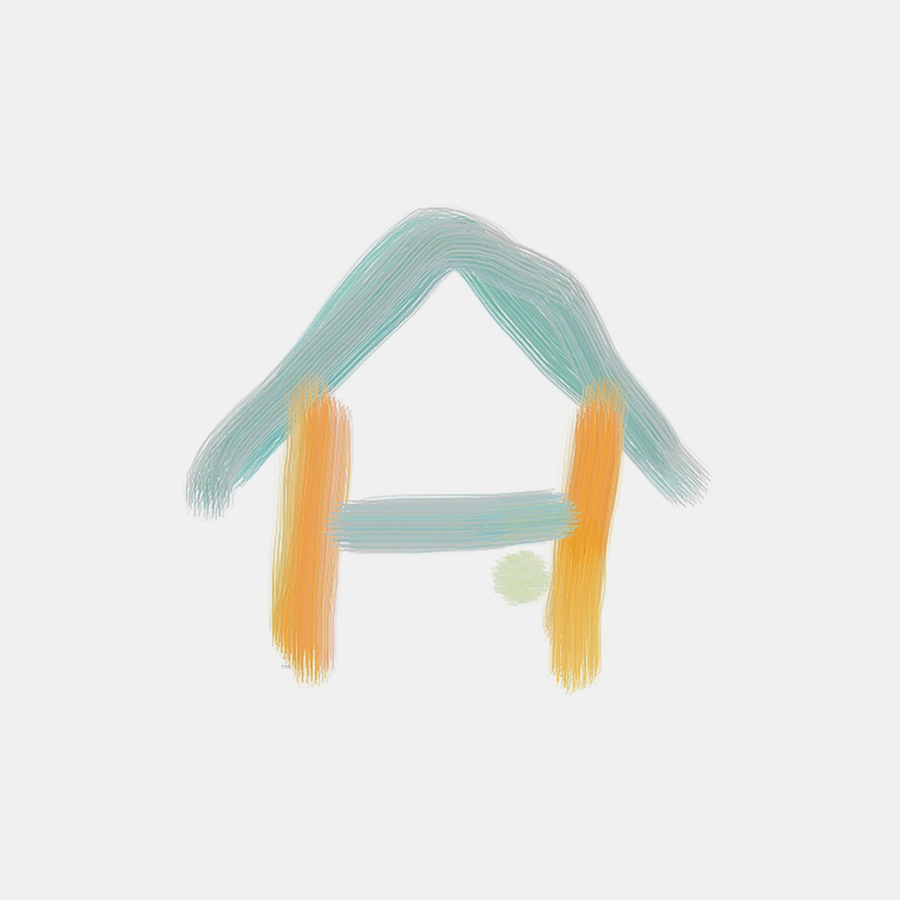Showing posts with label motif. Show all posts
Showing posts with label motif. Show all posts

### +walkA walk is some playable* spacetime complex process (sequence of finite steps at fixed lengths).** `Ludologically`, some progression and its contrapuntal motion.

This is a plot/flow of a nut's path (a type of circuit as it would traverse the braneworld, indicative of current) with stochastic activity at specified movements (). In all probability, walks chart a statistical distribution of their entropy (random variables transforming twistorspace).

In , there are three (3) stages to a walk: (1) coil, (2) graphics, and (3) mesh.

We use walks as parse arguments in juking. (see 🧑🏿lnq's walk, thread, sport, Pink program, route, tip, UUelcome)/// In automation, walks are approximate source directives.
Function map: preimagewalkimage

### +font

It is conjectured that Egglepple's family of fonts exist between amplitudes on a cryptograph. These motifs* are analogous to string tunings, and aid in the stereotypography of fibor.** Array of typefaces. (see font weight, font size, MONEY, fibor bundle, quantumquotient, UUelcome)Ludologically, as the definitive , we are said to find a font at the normalization of twistorspace where a dyad is an identity; that is, when an opus' measurable and permutable extrema commute (ie. idempotent yield deviation ≡ 0^).^^ That is, fret = 26¢ WITH `handicap` = \$676.00. (see foam)

Cryptologically, a font is the transactor between the supremum and the infimum of hashes where the resultant is optimal/most secure🔐 (ie. transaction with no tax).

According to jukebox operation (rotisserie), the complete count* of fonts (f) is simply ballet coordination in sesquilinear form, so f=1,352 [from encrypted (676) plus decrypted (676) classes] × 1.5 = 2,028.** Each `opus` `leaf` set contains three (3) fonts as a statistical mean./// A full sequencing of the `payload` (UUelcome) should reveal all motifs.
Function map: subchemistryfontchemistry || open stringfontknot || fontMONEY

### +fibor bundle

A fibor bundle (a function of taxation and/or ) is two (2) or more fibors combined/connected, which itself forms another fibor. This is Egglepple's quaternary isoform. (see bubblegum, hyperlink, minor scale, major scale, coverage, foam)Function map: minor scalemajor scalefiborfibor bundle

### +random coil

Ludologically, the function of opening🔓 a loopstring is processed seemingly random.
/// As in each outcome has equal probability.

A random coil is the lowest-level [dim≤1] (unmapped) orientation of a brane. This unstructured position sample [an algebraic curve] is known* as its preimage (or illustrative state for both pitch and incidental tax determinance).** I label it 'scribble'.

Instead of being a specific shape/pose, it is a statistical distribution of all populated conformations (albeit, strictly those lacking subunit definition). If each conformation (walk) has equal probability or weight, then such coil is the acoustically-approximated bounds of some twistorspace. (compare denaturation, contrast identity)
/// +It is not uncommon to have multiple different coil(s) for the same `opus/string`.
+The orientation is that of algebraic curvature because topologically, random coils are random walks on curved surfaces. My given axiom is that `encryption` can be hidden in its algebra.

Function map: scribblesponge
 🎮